Introduction

This tutorial demonstrates how sub-classes of the Node and QuadTree classes introduced in QuadTree: Python can be used to explore some aspects of blobby geometry. An example, of the interaction of positive and negative "blobby circles" is shown in figure 1. The quadtree (2D) technique shown in this tutorial is intended to be an introduction to 3D methods that use octrees.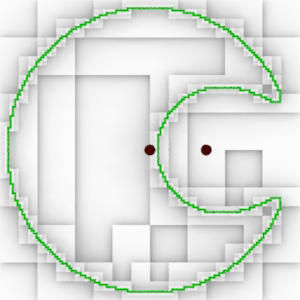Figure 1

Similar, but non-interacting, circles produced by cquadtree.py and visualized using RenderMan are shown in figure 2. For another tutorial that deals with blobby shapes refer to RSL: Blobby EffectsFigure 2

The Interaction of Positive & Negative Fields

Unlike figure 2 where the small green squares trace the circumferences of two circles, those in figure 1 mark the boundary of two "clouds" of (scalar) values such that their combined values are 0.5 or greater. The two dark red dots in figure 1 mark the centers of two radial fields of values that have a maximum value of 1.0 (positive field) and -1.0 (negative field) at the red dots and at a fixed radial distance have a value 0.0.

Figure 3 shows the positive and negative fields visualized as a grayscale. The green line marks locations where the grayscale value is 0.5.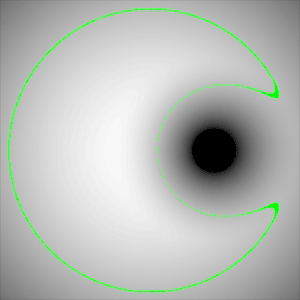Figure 3

Another way of visualizing a scalar field is to represent its values as heights - figure 4.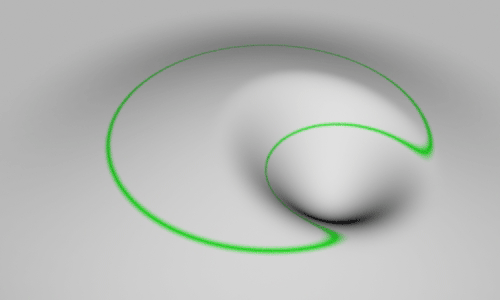Figure 4

The code presented later in this tutorial calculates the (scalar) field value at a point in space according to its distance from one or more blobby geometries such as circles and lines. The formula for converting a distance to a field value in the range 0 to 1 is taken from the research of Geoff Wyvill and Craig McPhetters "Data Structure for Soft Objects", The Visual Computer, Vol 2 1986. Wyvill calls his geometries Soft Objects. The terminology used in this tutorial follows Pixar's RenderMan and refers to Wyvill's soft objects as Blobby Geometries.

Others, such as Nishimura and Blinn name similar objects as Metaballs. For example, Nishimura et al "Object Modelling by Distribution Function and a Method of Image Generation", The Transactions of the Institute of Electronics and Communication Engineers of Japan, 1985, Vol. J68-D, Part 4, pp. 718-725, in Japanese, translated into English by Takao Fujuwara.

Wyvill & McPhetters

This tutorial, in an attempt to be as pictorial as possible, will not delve into the mathematics of their "field function". Different researchers have different ways of using distances to calculate a scalar field. Their technique is used here because it does not rely on ray tracing. Their technique is relatively fast and generates smooth iso-contours in 2D and smooth iso-surfaces in 3D. The prefix "iso" simply means "equal", as in a contour or a surface sharing the same field value. The code that performs the calculation is shown next.

 # Given the distance (squared) to the center of a circle or the # shortest distance to a line and the radius of influence (squared) # the proc returns a field value in the range 0 to 1. def fieldvalue(d2, r2): if d2 >= r2: return 0.0 d4 = d2 * d2 d6 = d2 * d4 r4 = r2 * r2 r6 = r2 * r4 return (-0.44444 * d6/r6 + 1.88889 * d4/r4 + -2.44444 * d2/r2 + 1)

Avoiding Sampling Errors

A quadtree subdivides a rectangle only if it detects that any of its four (children) sub-rectangles spans an iso-contour. Field values at each vertex might be calculated and if there is a mixture of values greater and smaller than 0.5 then the rectangle must be spanning an iso-contour (figure 5).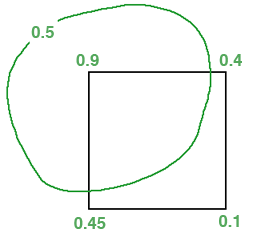Figure 5

Relying solely on vertex sampling will also fail if the iso-contour is completely contained within the rectangle (figure 6) or if the iso-contour only passes through an edge (figure 7).Figure 6Figure 7

 Figure 8 visualizes a quadtree that has relied solely on vertex sampling. Figure 9 demonstrates the use of a preliminary test that determines if the center of any of the blobby circles are with a sub-rectangle. Figure 10 shows the effect of applying a third test to determines if any of the edges of a sub-rectangle are within a certain distance of the center of any blobby circle. The tests are applied in the following order, from computationally cheapest to most expensive. A rectangle is forced to subdivide if,     it contains the center of a blobby circle,     the distance for any edge to a blobby circle is less than the radius/2,     its vertices have a mixture of field values < 0.5 and >= 0.5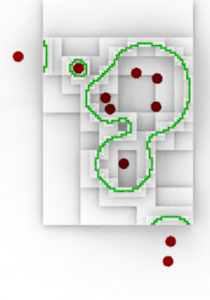Figure 8Figure 9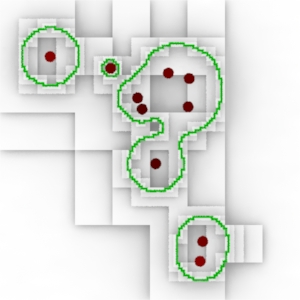Figure 10

 Because adjacent sub-rectangles share vertices their field values are stored in a dictionary that acts as a lookup table - see BlobbyNode.vertLUT. Previously calculated field values can then be reused by rectangles that share a vertex. The impact on memory useage is well worth the extra efficiency. For example, the quadtree for figure 11 was calculated in 400 milliseconds (Mac 2.66 GHz laptop). It reused 19,731 of the total 26,612 field value calculations. The same quadtree took 975 milliseconds without the use of the lookup table.Figure 11 The code in listing 2 should be saved in the same directory as quadtree.py, vectors.py and distances.py. Open blobby_quadtree.py with Cutter and look for the following comment, ### EDIT PATH ### Edit the path to the location where the archive rib will be saved. Use the keyboard shortcut control+e or alt+e to execute the script.